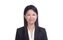cancel
Showing results for
Did you mean:Frequent Visitor

## Month-to-Month Change With Multiple Values

Hi all - i am trying to display month-to-month percentage for Multiple values (A, B, C) stored in the same colunm.

 Month IdAttribute Attribute Value June 1 A 200 June 2 B 100 June 3 C 200 July 1 A 50 July 2 B 25 July 3 C 500 August 1 A 30 August 2 B 250 August 3 C 115

Or

 A B C June 200 100 200 July 50 25 500 August 30 250 115

The value of B obtained in July is the result of the evolution of the value of A in June.
I would like to perform a Month/Month percentage calculation of B versus A and C versus B.
Simply: B (July)/A(June) = __% or C (July)/B(June) = __%

Do you have any tips (DAX measures) that could help me with this calculation?

2 ACCEPTED SOLUTIONSSuper User

Here is one way, .pbix file attachedFrequent Visitor

Hi

you need a common Date table, to use  PREVIOUSMONTH

total =SUM (value)

value_percent =

var a = SELECTEDVAUE (attribute)

return SWITCH (a,

"A", blank(),
"B", total / CALCULATE (total, REMOVEFILTER (attribute), attribute = "A", PREVIOUSMONTH (Date)),
"C", total / CALCULATE (total, REMOVEFILTER (attribute), attribute = "B", PREVIOUSMONTH (Date)),

blank() )

Creata Matrix with month and attribute like you show, and add "value_percent' as value

3 REPLIES 3Frequent Visitor

Hi

you need a common Date table, to use  PREVIOUSMONTH

total =SUM (value)

value_percent =

var a = SELECTEDVAUE (attribute)

return SWITCH (a,

"A", blank(),
"B", total / CALCULATE (total, REMOVEFILTER (attribute), attribute = "A", PREVIOUSMONTH (Date)),
"C", total / CALCULATE (total, REMOVEFILTER (attribute), attribute = "B", PREVIOUSMONTH (Date)),

blank() )

Creata Matrix with month and attribute like you show, and add "value_percent' as valueFrequent Visitor

However my main difficulty is that I would like to have a measure that works no matter which attribute is chosen, not only for B/A.
That is to say, a measure capable of producing the following result:

 A B C July B/A C/BSuper User

Here is one way, .pbix file attached